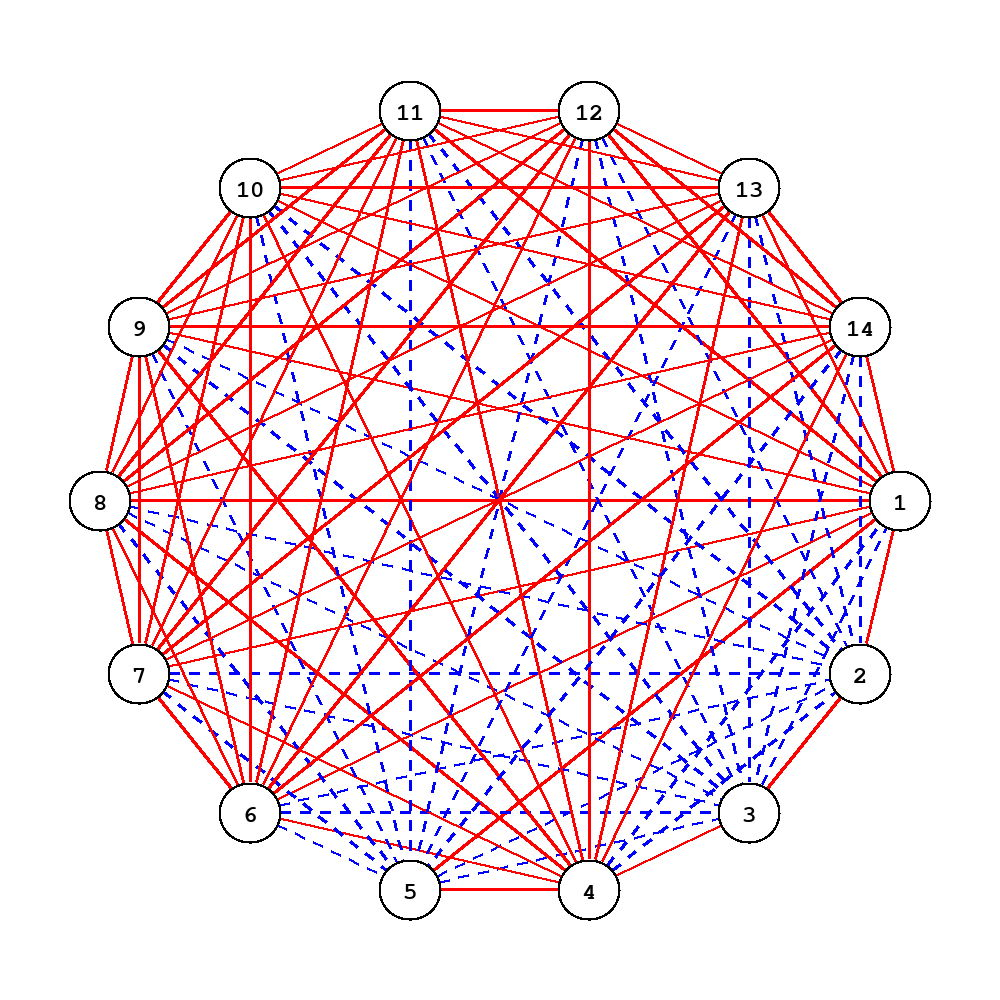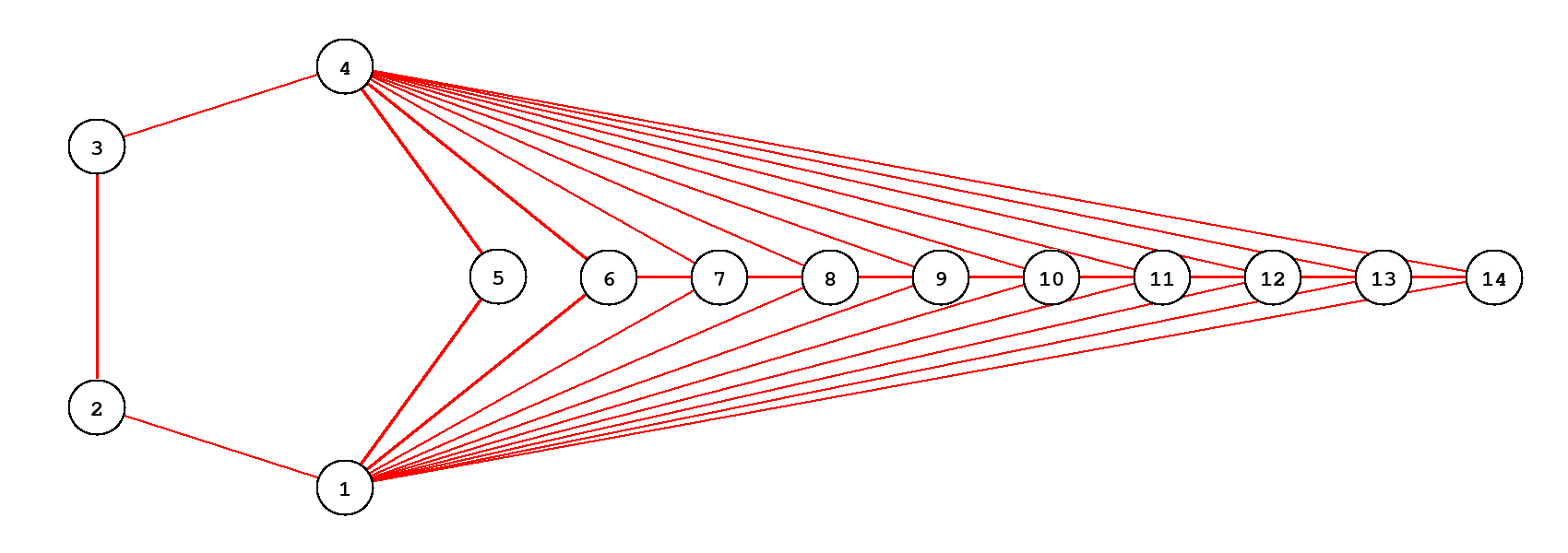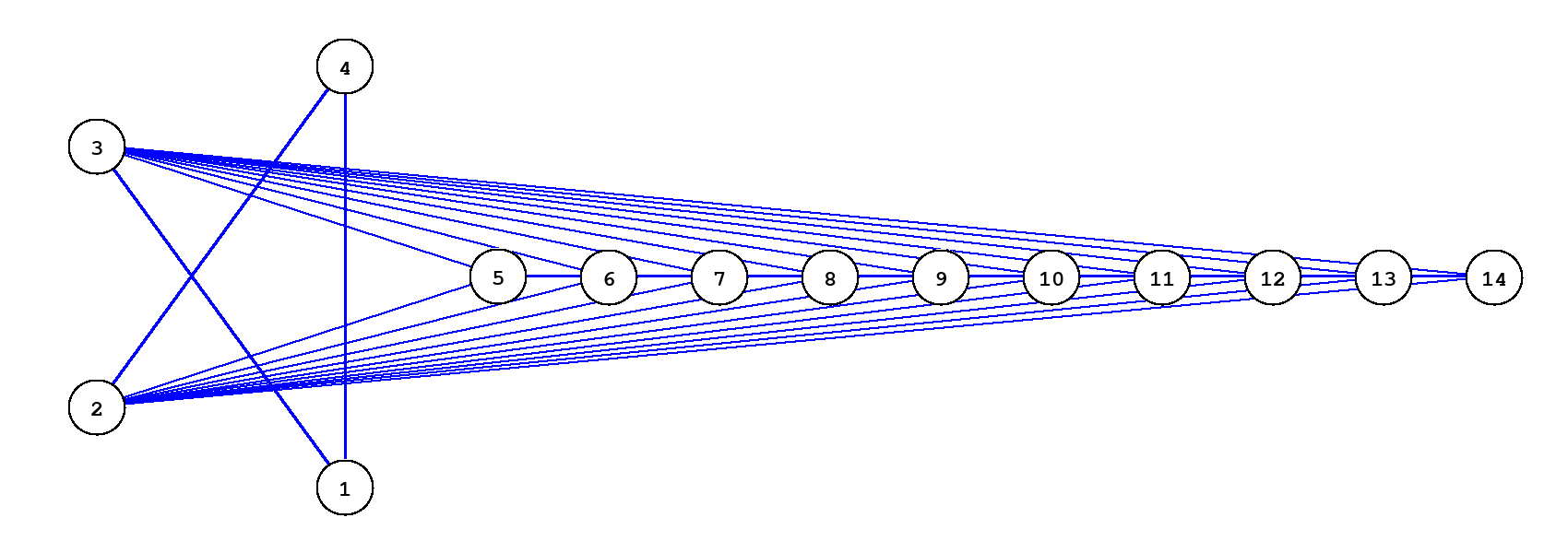시간 제한 메모리 제한 제출 정답 맞은 사람 정답 비율
2 초 256 MB 0 0 0 0.000%

## 문제

You are given a complete undirected graph with $7n$ vertices (here $n$ is a positive integer). Your task is to paint its edges in $n$ colors in such a way that for each pair of vertices and each color there is a path of at most two edges of this color connecting this pair of vertices. More formally, for each pair of vertices $u, v$ and each color $c$ at least one of the two options should hold:

• the edge between $u$ and $v$ has color $c$;
• there is a vertex $w$ that both edges $(u, w)$ and $(w, v)$ have color $c$.

## 입력

The only line of input contains a positive integer $n$ ($7\leqslant 7n\leqslant 1000$).

## 출력

Let us number the colors from $1$ to $n$. Let $c_{i, j}$ be $0$ if $i = j$, and the color of the edge $(i, j)$ in your coloring otherwise (in particular, in this case $c_{i, j} = c_{j, i}$). Print $c_{i, j}$ in $7n$ lines containing $7n$ numbers each.

It is guaranteed that a solution exists.

## 예제 입력 1

1


## 예제 출력 1

0 1 1 1 1 1 1
1 0 1 1 1 1 1
1 1 0 1 1 1 1
1 1 1 0 1 1 1
1 1 1 1 0 1 1
1 1 1 1 1 0 1
1 1 1 1 1 1 0


## 예제 입력 2

2


## 예제 출력 2

0 1 2 2 1 1 1 1 1 1 1 1 1 1
1 0 1 2 2 2 2 2 2 2 2 2 2 2
2 1 0 1 2 2 2 2 2 2 2 2 2 2
2 2 1 0 1 1 1 1 1 1 1 1 1 1
1 2 2 1 0 2 2 2 2 2 2 2 2 2
1 2 2 1 2 0 1 1 1 1 1 1 1 1
1 2 2 1 2 1 0 1 1 1 1 1 1 1
1 2 2 1 2 1 1 0 1 1 1 1 1 1
1 2 2 1 2 1 1 1 0 1 1 1 1 1
1 2 2 1 2 1 1 1 1 0 1 1 1 1
1 2 2 1 2 1 1 1 1 1 0 1 1 1
1 2 2 1 2 1 1 1 1 1 1 0 1 1
1 2 2 1 2 1 1 1 1 1 1 1 0 1
1 2 2 1 2 1 1 1 1 1 1 1 1 0


## 힌트

The second sample test corresponds to the following coloring:Here are two separate subgraphs for both colors: